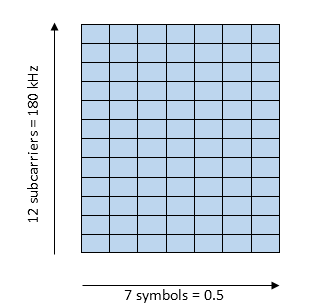The theoretical calculation for the LTE Air Interface is as follows

One Resource block(RB) can have 12 subcarriers (each carrier is 15 kHz) in frequency domain and 0.5ms (7 symbols) in time domain.Thus, total number of symbols per resource block = 12 * 7 = 84

Each symbol can accommodate certain number of bits based on the modulation scheme as per the table below

 Modulation scheme Bits per symbol QPSK 2 16-QAM 4 64-QAM 6

The table below shows the number of resource blocks available for different LTE channel bandwidths.

 Channel Bandwidth (MHz) 5 10 15 20 Resource Blocks (RB) 25 50 75 100 No. of subcarriers (RB*12) 300 600 900 1200 Occupied bandwidth (MHz) 4.5 9 13.5 18

Note: In LTE 10% of total bandwidth is used for guard band. For example if the channel bandwidth is 20MHz, then 2MHz is used for guard band. Thus, if 80 kHz has 1 RB, 18 MHz will have 100 RBs

Given below is the calculation for PHY rate:

In LTE for 20MHz, there are 100 Resource blocks and each Resource block has 12*7 = 84 symbols

Example: PHY rate calculation for 20MHz band, using 64-QAM and 4*4 Tx Rx antennae

• For 20 MHz there are 100 Resource blocks
• Each resource block has 12*7 = 84 symbols
• 100 resource blocks have 8400 symbols
• 1 sub frame = 1 ms = 2 time slots
• 16800 symbols per subframe (or per ms)
• 64-QAM can transmit 6 bits per symbol
• 1 subframe using 64-QAM modulation can transmit
• 100.8*10^6 bits per second or 100.6 Mbps
• This is for a 1*1 Tx Rx antenna and for 4*4 Tx Rx antennae 100.8*4 = 403.2 Mbps

The above note explains the theoretical method of calculating the LTE PHY Data rate, where there are no channel (propagation) losses.

However in the real world there is signal attenuation due to propagation losses. Thus the calculation for PHY Data rate in NetSim is based on the Transport Block Size which is specified in the standard. This calculation is as follows

1. Any signal received at the receiver has a SNR (signal to noise ratio).

2. Based on the SNR a CQI value is calculated.

3. The SNR - CQI Table is available in LTE.h in NetSim and is per the LTE standard

4. Based on the SNR and the CQI an MCS value is calculated

5. The SNR CQI MCS table is available in LTE.h in NetSim and is per the LTE standard

6. Based on the MCS the TBS Index is calculated, again from a table available in LTE.h which is per the LTE Standard

7. Based on the TBS Index the TBS Table is looked up and the transport block size is retrieved

Approximately 25% of overhead used for controlling and signalling. Effective PHY data rate is 300 Mbps